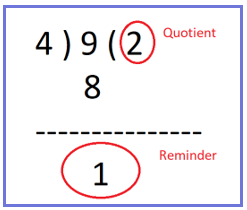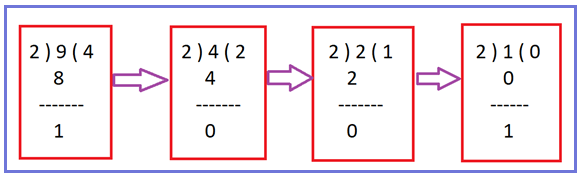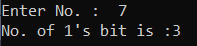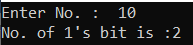# Count Number of 1 Bit in a Given Number in C#

## Count Number of 1 Bit in a Given Number in C# with Examples

In this article, I am going to discuss How to Count the Number of 1 Bit in a given number in C# with Examples. Please read our previous article, where we discussed How to Check whether a number is a Power of 2 or not in C#. Given an integer find the number of 1 bit it has.

Example1:
Input: 6 (Binary representation of 6 is 110)
Output: 2
Explanation: 110 has 2 numbers of 1 bit.

Example2:
Input: 7 (Binary representation of 7 is 111)
Output: 3
Explanation: 111 has 3 numbers of 1 bit.

We are going to use the very basic methods to implement this program but before that, you should have some basic knowledge of the following operators.

1. Modulus (%) Operator.
2. Divide (/) Operator.

Modulus %: Modulus Operator will return a reminder of the solution as result.
Example: 97 % 10 = 7
43 % 10 = 3

Divides /: Divides Operator will return the quotient of the solution as result.
Example: 97 / 10 = 9
43 / 10 = 4##### Logic to Count Number of 1 Bit in C#
1. Take the input from the user in a variable called no.
2. If the entered number is smaller than or equal to 0 throw an error message.
3. Otherwise, take another variable called count to store the value of No. of 1’s present in the number.
4. Then Module the number with 2 and if it is not equal to 0 increments the value of count by 1.
5. Then Divide the number by 2 and store the value in the same variable named no.
6. Repeat the above two steps till the number is greater than 0.
7. At the end print the value of the count variable as the no. of 1’s present in the digit.##### Algorithm to Count Number of 1 Bit

Step1: Take input from the user and store this into no.
Step2: Take a variable named count and initialize it with 0.
Step3: Check if no is less than or equal to 0 then throw an exception “No should be greater than 0”.
Step4: If No % 2 !=0 then increment the value of the count variable by 1.
If(no % 2 != 0)
count++;
Step5: Store the result of no/2 into no.
no = no / 2;
Step6: Repeat steps 4 and 5 until no is greater than 0.
Step7: Show the value of count as No of bits as output.

###### Example: C# program to count no of 1 bit in a number.

The following C# Program will count the number of 1 bit in a given number.

```using System;
namespace DotNetTutorials
{
public class CountNoOf1Bits
{
static void Main(string[] args)
{
try
{
Console.Write("Enter No. :  ");
int count = 0;
if (no <= 0)
throw new Exception("No. should be greater than 0");

while (no > 0)
{
if (no % 2 != 0)
count++;
no /= 2;
}
Console.WriteLine("No. of 1's bit is :" + count);
}
catch (Exception ex)
{
Console.WriteLine(ex.Message);
}
}
}
}
```
###### Output:##### C# Code (Using function)

In the below C# example, we have created one method to count the number of 1 bit for a given number.

```using System;
namespace DotNetTutorials
{
public class CountNoOf1Bits
{
static void Main(string[] args)
{
try
{
Console.Write("Enter No. :  ");
int count = Count1Bit(no);
Console.WriteLine("No. of 1's bit is :" + count);
}
catch (Exception ex)
{
Console.WriteLine(ex.Message);
}
}

private static int Count1Bit(int no)
{
int count = 0;
if (no <= 0)
throw new Exception("No. should be greater than 0");

while (no > 0)
{
if (no % 2 != 0)
count++;
no /= 2;
}

return count;
}
}
}
```
###### Output:In the next article, I am going to discuss How to Count the Number of Digits in an integer in C# with Examples. Here, in this article, I try to explain How to find the number of 1 bit in a given number in C# with Examples and I hope you enjoy this How to find the number of 1 bit in a given number in the C# article.